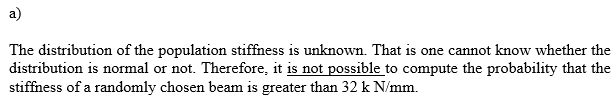# The stiffness of a certain type of steel beam used in building construction has mean 30 kN/mm and standard deviation 2 kN/mm. a) Is it possible to compute the probability that the stiffness of a randomly chosen beam is greater than 32 kN/mm? If so, compute the probability. If not, explain why not. b) In a sample of 100 beams, is it possible to compute the probability that the sample mean stiffness of the beams is greater than 30.2 kN/mm? If so, compute the probability. If not, explain why not.

Question
1 views

The stiffness of a certain type of steel beam used in building construction has mean 30 kN/mm and standard deviation 2 kN/mm. a) Is it possible to compute the probability that the stiffness of a randomly chosen beam is greater than 32 kN/mm? If so, compute the probability. If not, explain why not. b) In a sample of 100 beams, is it possible to compute the probability that the sample mean stiffness of the beams is greater than 30.2 kN/mm? If so, compute the probability. If not, explain why not.

check_circle

Step 1...

### Want to see the full answer?

See Solution

#### Want to see this answer and more?

Solutions are written by subject experts who are available 24/7. Questions are typically answered within 1 hour.*

See Solution
*Response times may vary by subject and question.
Tagged in